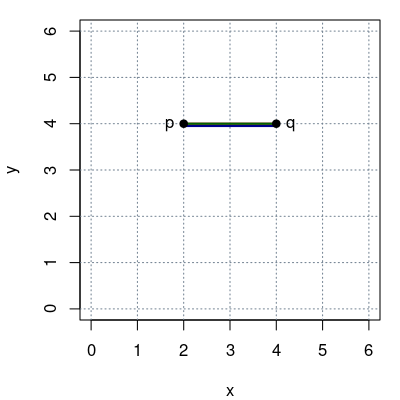## Exam 1

1. #### Question

Given two points $p = (2, 4)$ and $q = (4, 4)$ in a Cartesian coordinate system:

1. What is the Manhattan distance $d_1(p, q)$?
2. What is the Euclidean distance $d_2(p, q)$?
3. What is the maximum distance $d_\infty(p, q)$?

#### Solution

The distances are visualized below in green ($d_1$), red ($d_2$), and blue ($d_\infty$).1. $d_1(p, q) = \sum_i |p_i - q_i| = |2 - 4| + |4 - 4| = 2$.
2. $d_2(p, q) = \sqrt{\sum_i (p_i - q_i)^2} = \sqrt{(2 - 4)^2 + (4 - 4)^2} = 2$.
3. $d_\infty(p, q) = \max_i |p_i - q_i| = \max(|2 - 4|, |4 - 4|) = 2$.